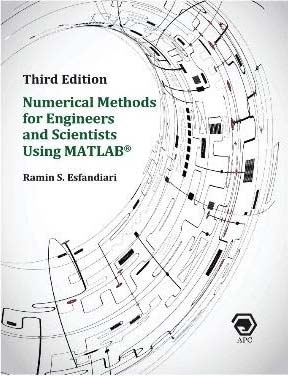## Inside: Numerical Methods for Engineers and Scientists Using MATLAB® 3rd EditionNumerical Methods for Engineers and Scientists Using MATLAB®3rd Edition

# Preface

The primary goal of this book is to provide the reader with a broad knowledge of the fundamentals of numerical methods utilized in various disciplines in engineering and science. The powerful software MATLAB®s introduced at the outset and is assimilated throughout the book to perform symbolic, graphical, and numerical tasks. The textbook, written at the junior/senior level, methodically covers a wide range of techniques ranging from curve fitting a set of data to numerically solving initial- and boundary-value problems. Each method is accompanied by at least one fully worked-out example, followed by either a user-defined function or a MATLAB script file.

The book consists of 10 chapters. Chapter 1 presents an in-depth introduction to the essentials of MATLAB as related tonumerical methods. The chapter addresses fundamental features such as built-in functions and commands, formattingoptions, vector and matrix operations, program flow control, symbolic operations, and plotting capabilities. The readeralso learns how to write a user-defined function or a MATLAB script file to perform specific tasks.

Chapter 2 covers introduction to numerical methods. This includes sources of computational error, approximations,iterative methods, and rates of convergence.

Chapters 3 and 4 introduce numerical methods for solving equations. Chapter 3 focuses on finding roots of equations ofa single variable, while Chapter 4 covers methods for solving linear and nonlinear systems of equations.

Chapter 5 is devoted to curve fitting and interpolation techniques. Chapter 6 discusses numerical differentiation andintegration methods. Chapters 7 and 8 present numerical methods for solving initial-value problems and boundary-valueproblems, respectively.

Chapter 9 covers the numerical solution of the matrix eigenvalue problem, which entails techniques to approximate a fewor all eigenvalues of a matrix. Chapter 10 presents numerical methods for solving elliptic, parabolic and hyperbolic partial differential equations, specifically those that frequently arise in engineering and science.

## New to This Edition

• Additional details on the presentation of material
• Increased display of hand calculations in the worked-out examples
• Updating the MATLAB codes and functions based on a more recent release (R2021a Release)

## Pedagogy of the Book

The book is very user-friendly, in an effort to make the material easy to understand by the reader. The following format is utilized throughout the book:

• Each method is accompanied by at least one fully worked-out example showing all details
• This is followed by a user-defined function, or a script file, that utilizes the method to perform a desired task
• The hand calculations are then confirmed through the execution of the user-defined function or the script file
• Whenever possible, MATLAB built-in functions are executed for re-confirmation of the results
• Plots are regularly generated to shed light on the accuracy and significance of the numerical results

## Exercises

A large set of problems/projects with various levels of difficulty is included at the end of each chapter, which can be
worked out using either

• Hand calculation, or
• MATLAB ®

In many instances, the reader is asked to prepare a user-defined function or a script file that implements a specific
technique, which often require revisions to those already presented in the chapter.